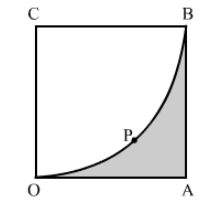# In the given figure, OABC is a square of side 7 cm.`
Question:

In the given figure, OABC is a square of side 7 cm. If COPB is a quadrant of a circle with centre C find the area of the shaded region.Solution:

Area of shaded region = Area of square OABC − Area of quadrant COPB having radius OC

$=(\text { Side })^{2}-\frac{1}{4}\left(\pi \times r^{2}\right)$

$=(7)^{2}-\frac{1}{4}\left[\frac{22}{7} \times 7^{2}\right]$

$=49-38.5$

$=10.5 \mathrm{~cm}^{2}$

Hence, the area of the shaded region is 10.5 cm2